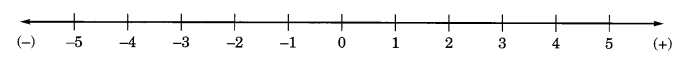On this page, you will find Whole Numbers Class 6 Notes Maths Chapter 2 Pdf free download. CBSE NCERT Class 6 Maths Notes Chapter 2 Whole Numbers will seemingly help them to revise the important concepts in less time.

## CBSE Class 6 Maths Chapter 2 Notes Whole Numbers

### Whole Numbers Class 6 Notes Conceptual Facts

1. The numbers 1, 2, 3, 4, 5, … which are used for counting are known as natural numbers. They are represented by N.

2. All natural numbers together with zero are called whole numbers. They are represented by W.
So, W = 0, 1, 2, 3, 4, …

3. Every natural number is a whole number but reverse not true.

4. 0 is the smallest whole number whereas 1 is the smallest natural number.

5. We have no largest natural number or whole number.

6. The successor of a whole number is 1 more than the whole number.

7. The predecessor of a whole number is 1 less than the whole number but 0 has no predecessor.

8. All the natural numbers and whole numbers can be represented on number line.

9. On number line, positive numbers are taken on right side of the zero and negative numbers on left side.Properties of whole numbers

If a, b, e are any whole numbers, then

• Closure property for addition: a + b is a whole number.
• Closure property for multiplication: a x b is a whole number.
• Commutative property for addition: a + b = b + a.
• Commutative property for multiplication: a x b = b x a
• Associative property for addition: a + (b + c) = (a + b) + c
• Associative property for multiplication: a x (b x c) = (a x b) x c
• Distributive property: a x (b + c) = a x b + a x c
• Identity for addition: a+0 = 0+a=a
• Identity for multiplication: a x 1 = 1 x a=a
• 0 ÷ a = 0 but a ÷ 0 is not defined.